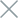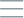• Home
• Tags
• Series
•# Random Search Algorithm in Java: Introduction, How It Works, Example

The Random Search algorithm, also known as Monte Carlo search, is a searching method based on randomness. Instead of sequentially checking each element in a data array, this algorithm randomly selects a number of elements to examine. This approach saves time and resources compared to sequential searching.

## How It Works

1. Step 1: Begin with the data array you want to search.

2. Step 2: Randomly select a certain number of elements to examine.

3. Step 3: Check the selected elements to see if they match the search condition.

4. Step 4: If a matching element is found, return the result; if not, return to Step 2.

5. Step 5: Continue the process until a match is found or the maximum number of attempts is reached.

• Resource-Efficient: Saves time and memory, especially for large data arrays.
• Randomness: Not easily predictable, suitable for situations that require randomness.

• No Guarantee of Success: There is no assurance that the algorithm will find the desired result.
• May Take a Long Time: In the worst case, the algorithm can take longer than sequential search.

## Example and Explanation

Consider the following example of using the Random Search Algorithm to find an integer in an array:

``````import java.util.Random;

public class RandomSearchExample {
static int randomSearch(int[] arr, int target) {
Random rand = new Random();
int maxAttempts = arr.length; // Maximum number of attempts
for (int i = 0; i < maxAttempts; i++) {
int randomIndex = rand.nextInt(arr.length); // Randomly select an index
if (arr[randomIndex] == target) {
return randomIndex; // Return the index if found
}
}
}

public static void main(String[] args) {
int[] numbers = {1, 5, 9, 3, 7};
int target = 3;
int result = randomSearch(numbers, target);
if (result != -1) {
System.out.println("Number " + target + " found at index " + result);
} else {